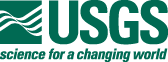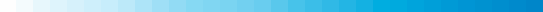Virginia Aeromagnetic Data ProcessingThe assembly of the 23 individual aeromagnetic surveys and grids to create the Virginia state-wide compilation was done in several steps using in-house software (Phillips, 1997).

DATA PROCESSING STEPS

1. Grids were constructed from the original aeromagnetic survey data with a cell size of between 1/3 and 1/5 of the flight-line spacing of the survey, using a minimum curvature gridding algorithm (Webring, 1981). For digitized contour line data, the initial grid was constructed using a minimum curvature algorithm and a spacing appropriate for the scale of the digitized map.
2. The Definitive Geomagnetic Reference Field (DGRF) calculated for the date and elevation of the original survey was subtracted. If an obsolete regional field other than the DGRF had been removed, as was the case with much of the digitized data, the original geomagnetic reference field was added back and the DGRF (Sweeney, 1990) was subtracted from the grid.
3. The original survey grids were continued to 500 ft (152.4 m) above ground and converted from level to drape as necessary. Upward continuation of surveys flown at elevations less than 500 ft (152.4 m) above ground was accomplished using standard 2D Fast Fourier Transform (FFT) filtering techniques (Hildenbrand, 1983). Surveys flown at altitudes greater than 500 ft (152.4 m) were continued downward using equivalent source techniques (Phillips, 1996, Xia and others, 1993).
4. The survey grids were regridded, as necessary, to the final grid cell size of 500 m using a minimum curvature algorithm.
5. The datum levels of the converted grids were adjusted (by addition or subtraction of a constant value) to minimize differences at the boundaries.
6. These adjusted grids were combined into a single merged grid that shows the magnetic field measured or calculated at 500 ft (152.4 m) above ground.
7. The final merged grid was continued upward to 1000 ft (304.8 m) above ground using the Oasis Montaj software package by Geosoft. This level was selected in order to be compatible with recent USGS state compilations.

GRID PROJECTION SPECIFICATIONS

• Projection = Lambert conformal conic
• Central meridian = 79 degrees W
• Base latitude = 0 degrees N
• Standard parallels = 33 and 45 N
• Semi-major ellipsoid axis = 6378206.4 m
• Eccentricity squared = 0.0067686579973
• Ellipsoid = Clarke 1866